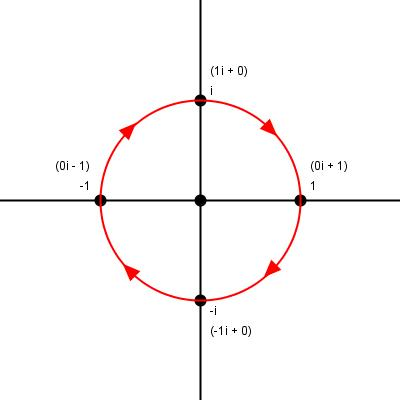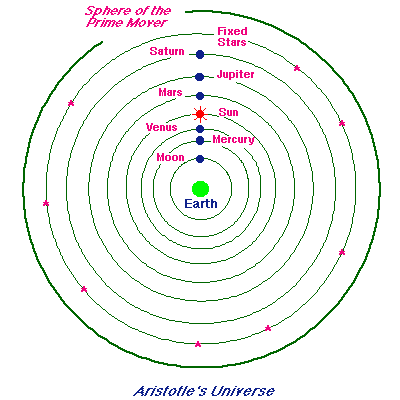# Difference between Ellipse and Oval

Although an oval and ellipse look very similar, there are many few differences between these two shapes in geometry. It is extremely important to understand the appropriate meaning of these two figures to avoid confusion. The smooth curves and similarities to a circle, an oval and an ellipse are almost identical. Here are some instructions and tips to learn the key differences between these two shapes. When an intersection of the plane surface and the conic surface takes place, a closed curve is formed which is also known as an ellipse. Although an oval is a not a recognised geometrical figure in mathematics but just like an ellipse, it is a circle stretched over two ends.

### Instructions

• 1

Ellipse:

An ellipse simply has an eccentricity between one and zero. On the other hand, the focus of the set of points on a mathematical plane is also known an ellipse. However, for this definition to be true, the sum of distances from two fixed points to the point must be constant. In mathematics, the distance between the two fixed points and the focal point is called the foci. Usually a string is tied to the two fixed points when drawing an ellipse on a piece of paper. The line that passes through these two fixed points is known as the major axis. Another line passing through the center of the ellipse is called the minor axis, which is always perpendicular to the major axis. The diameter for major axis knows as the transverse diameter.

Diameter for the minor axis, on the other hand, is termed as the conjugate diameter. Similarly part of the main axis is called the semi major axis and part of the minor axis is known as the semi minor axis. The figure of an ellipse plays a key role in a number of mathematical applications. Physics in particular uses the rules of an ellipse to solve complex problems. One of the applications of an ellipse is the orbits of planets in the universe. Furthermore, the reflectors for acoustic devices and antennas are also created in this shape to ensure converged focus.

- Image courtesy: elegantcoding.com• 2

Oval:

As discussed before, an oval is not considered a shape in mathematics. Just like an ellipse, it resembles the shape of an egg. All ellipses are oval but not all ovals are ellipses. Ovals usually have at least one axis of symmetry and have properties including simple, convex closed plane curves and smooth.

- Image courtesy: nakkeran.com•